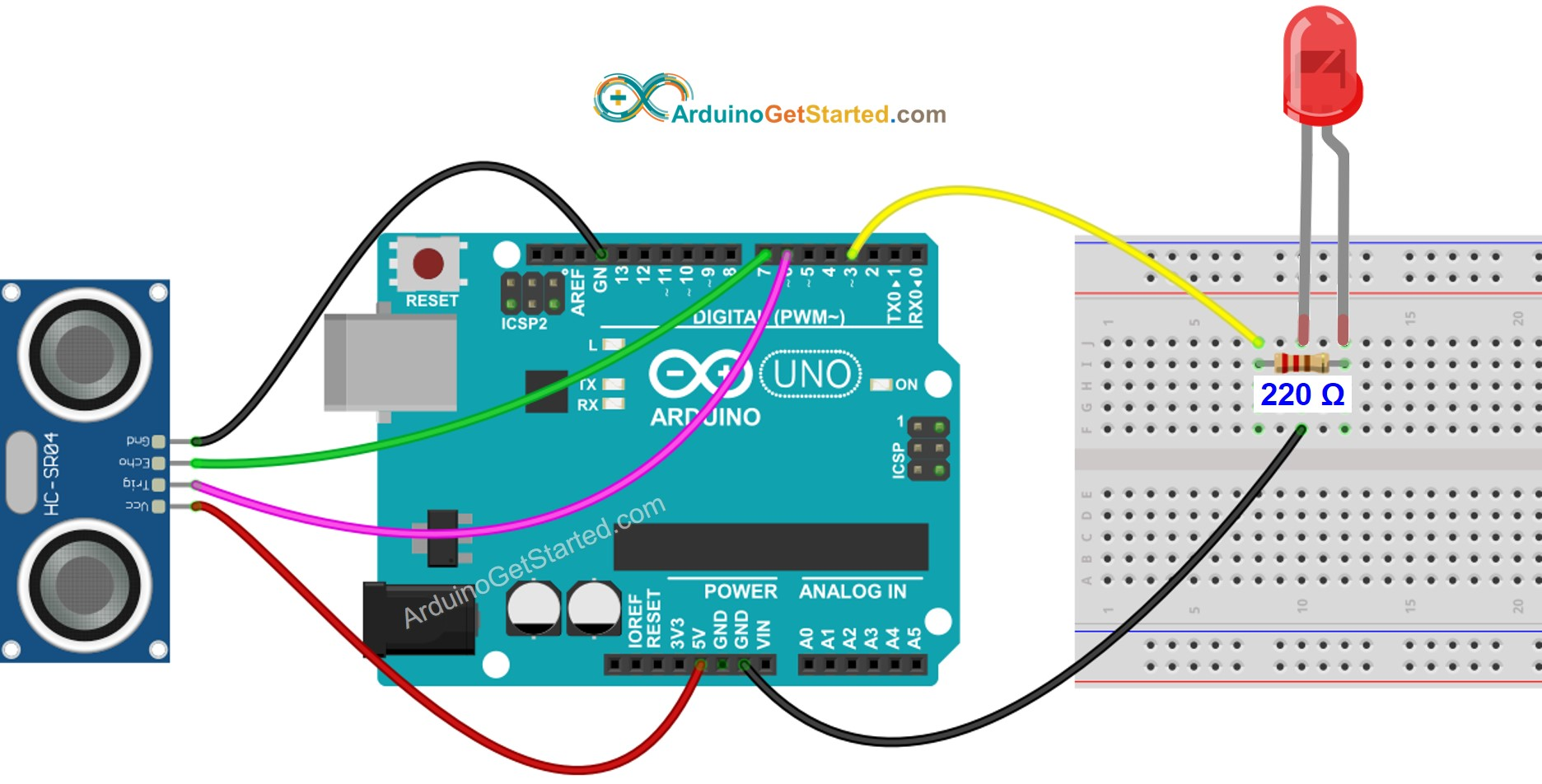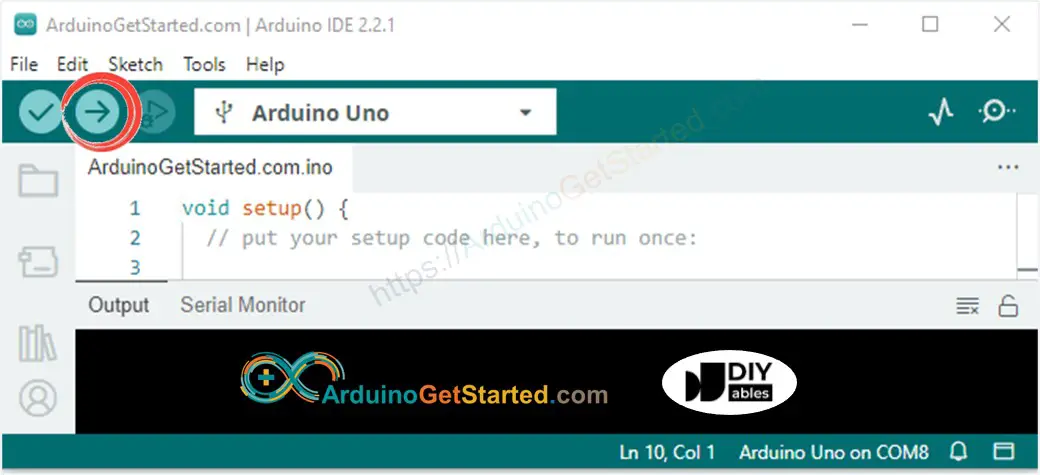# Arduino - Ultrasonic Sensor - LED

We are going to learn how to:

• If the object is close to ultrasonic sensor, turn LED on
• If the object is far from ultrasonic sensor, turn LED off

## Hardware Required

Please note: These are affiliate links. If you buy the components through these links, We may get a commission at no extra cost to you. We appreciate it.

## About LED and Ultrasonic Sensor

If you do not know about LED and ultrasonic sensor (pinout, how it works, how to program ...), learn about them in the following tutorials:

## Wiring DiagramImage is developed using Fritzing. Click to enlarge image

## Arduino Code

/* * Created by ArduinoGetStarted.com * * This example code is in the public domain * * Tutorial page: https://arduinogetstarted.com/tutorials/arduino-ultrasonic-sensor-led */ // constants won't change const int TRIG_PIN = 6; // Arduino pin connected to Ultrasonic Sensor's TRIG pin const int ECHO_PIN = 7; // Arduino pin connected to Ultrasonic Sensor's ECHO pin const int LED_PIN = 3; // Arduino pin connected to LED's pin const int DISTANCE_THRESHOLD = 50; // centimeters // variables will change: float duration_us, distance_cm; void setup() { Serial.begin (9600); // initialize serial port pinMode(TRIG_PIN, OUTPUT); // set arduino pin to output mode pinMode(ECHO_PIN, INPUT); // set arduino pin to input mode pinMode(LED_PIN, OUTPUT); // set arduino pin to output mode } void loop() { // generate 10-microsecond pulse to TRIG pin digitalWrite(TRIG_PIN, HIGH); delayMicroseconds(10); digitalWrite(TRIG_PIN, LOW); // measure duration of pulse from ECHO pin duration_us = pulseIn(ECHO_PIN, HIGH); // calculate the distance distance_cm = 0.017 * duration_us; if(distance_cm < DISTANCE_THRESHOLD) digitalWrite(LED_PIN, HIGH); // turn on LED else digitalWrite(LED_PIN, LOW); // turn off LED // print the value to Serial Monitor Serial.print("distance: "); Serial.print(distance_cm); Serial.println(" cm"); delay(500); }

### Quick Steps

• Connect Arduino to PC via USB cable
• Open Arduino IDE, select the right board and port
• Copy the above code and open with Arduino IDE
• Click Upload button on Arduino IDE to upload code to Arduino
•• Move your hand in front of sensor
• See the change of LED's state

### Code Explanation

Read the line-by-line explanation in comment lines of source code!

## Video Tutorial

We are considering to make the video tutorials. If you think the video tutorials are essential, please subscribe to our YouTube channel to give us motivation for making the videos.

※ OUR MESSAGES